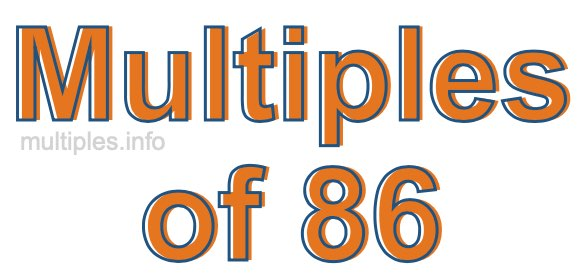Multiples of 86Welcome to the Multiples of 86 page. Here we will first teach you everything you will ever need to know about the multiples of 86, and then give you a study guide summary of everything we taught you to make sure you remember it all. Use this page to look up facts and learn information about the multiples of 86. This page will make you a multiples of eighty-six expert!

Definition of Multiples of 86
Multiples of 86 are all the numbers that when divided by 86 equal an integer. Each of the multiples of 86 are called a multiple. A multiple of 86 is created by multiplying 86 by an integer.

Therefore, to create a list of multiples of 86, you start with 1 multiplied by 86, then 2 multiplied by 86, then 3 multiplied by 86, and so on for as long as you want. Thus, the list of the first five multiples of 86 is 86, 172, 258, 344, and 430. To see a larger list of multiples of 86, see the printable image of Multiples of 86 further down on this page. We also have a category where you can choose any nth multiple of 86.

Multiples of 86 Checker
The Multiples of 86 Checker below checks to see if any number of your choice is a multiple of 86. In other words, it checks to see if there is any number (integer) that when multiplied by 86 will equal your number. To do that, we divide your number by 86. If the the quotient is an integer, then your number is a multiple of 86.

Is  a multiple of 86?

Least Common Multiple of 86 and ...
A Least Common Multiple (LCM) is the lowest multiple that two or more numbers have in common. This is also called the smallest common multiple or lowest common multiple and is useful to know when you are adding our subtracting fractions. Enter one or more numbers below (86 is already entered) to find the LCM.

Check out our LCM Calculator if you need more details about the Least Common Multiple or if you need the LCM for different numbers for adding and subtraction fractions.

nth Multiple of 86
As we stated above, 86 is the first multiple of 86, 172 is the second multiple of 86, 258 is the third multiple of 86, and so on. Enter a number below to find the nth multiple of 86.

th multiple of 86

Multiples of 86 vs Factors of 86
86 is a multiple of 86 and a factor of 86, but that is where the similarities end. All postive multiples of 86 are 86 or greater than 86. All positive factors of 86 are 86 or less than 86.

Below is the beginning list of multiples of 86 and the factors of 86 so you can compare:

Multiples of 86: 86, 172, 258, 344, 430, etc.

Factors of 86: 1, 2, 43, 86

As you can see, the multiples of 86 are all the numbers that you can divide by 86 to get a whole number. The factors of 86, on the other hand, are all the whole numbers that you can multiply by another whole number to get 86.

It's also interesting to note that if a number (x) is a factor of 86, then 86 will also be a multiple of that number (x).

Multiples of 86 vs Divisors of 86
The divisors of 86 are all the integers that 86 can be divided by evenly. Below is a list of the divisors of 86.

Divisors of 86: 1, 2, 43, 86

The interesting thing to note here is that if you take any multiple of 86 and divide it by a divisor of 86, you will see that the quotient is an integer.

Multiples of 86 Table
Below is an image of the first 100 multiples of 86 in a table. The table is in chronological order, column by column. The first column has the first ten multiples of 86, the second column has the next ten multiples of 86, and so on.The Multiples of 86 Table is also referred to as the 86 Times Table or Times Table of 86. You are welcome to print out our table for your studies.

Negative Multiples of 86
Although not often discussed or needed in math, it is worth mentioning that you can make a list of negative multiples of 86 by multiplying 86 by -1, then by -2, then by -3, and so on, to get the following list of negative multiples of 86:

-86, -172, -258, -344, -430, etc.

Multiples of 86 Summary
Below is a summary of important Multiples of 86 facts that we have discussed on this page. To retain the knowledge on this page, we recommend that you read through the summary and explain to yourself or a study partner why they hold true.

There are an infinite number of multiples of 86.

A multiple of 86 divided by 86 will equal a whole number.

86 divided by a factor of 86 equals a divisor of 86.

The nth multiple of 86 is n times 86.

The largest factor of 86 is equal to the first positive multiple of 86.

86 is a multiple of every factor of 86.

86 is a multiple of 86.

A multiple of 86 divided by a divisor of 86 equals an integer.

86 divided by a divisor of 86 equals a factor of 86.

Any integer times 86 will equal a multiple of 86.

Multiples of a Number
Here you can get the multiples of another number, all with the same attention to detail as we did for multiples of 86 on this page.

Multiples of
Multiples of 87
Did you find our page about multiples of eighty-six educational? Do you want more knowledge? Check out the multiples of the next number on our list!

Copyright  |   Privacy Policy  |   Disclaimer  |   Contact